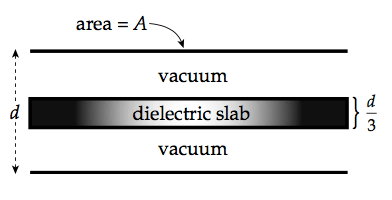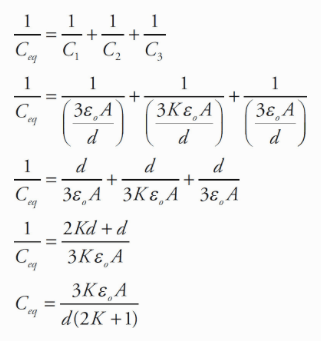# Question 41

• N=m·kg·s-2

• J=m2·kg·s-2

• C=A·s

• V=m2·kg·s-3A-1

• T=kg·s-2A-1

# Question 48

•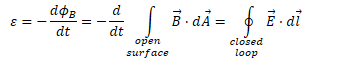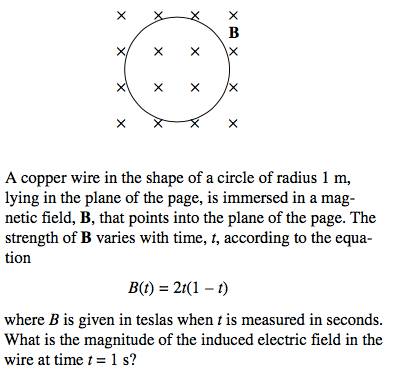# Question 53

• Parallel

capacitors:

•# Question 56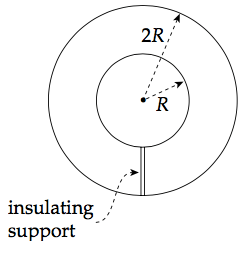• Calculating Capacitance

1. Assume a charge of +Q and -Q on each conductor

2. Find the electric field between the conductors (Gauss's Law)

•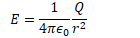3.•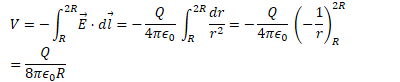4.•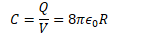# Question 57

• Since the magnetic force is always perpendicular to the object’s velocity, it does zero work on any charged particle.

• Zero work means zero change in kinetic energy, so the speed remains the same.

• Remember: The magnetic force can only change the direction of a charged particle’s velocity, not its speed.

# Question 61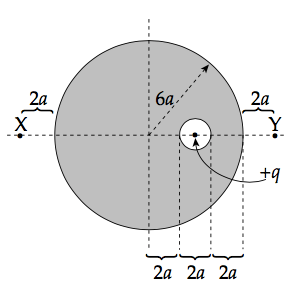![If a conducting sphere contains a charge of + q within an inner cavity, a charge of —q will move to the wall of the cavity to "guard" the inte- rior of the sphere from an electrostatic field, regardless of the size, shape, or location of the cavity. As a result, a charge of +q is left on the exterior of the sphere (and it will be uniform). So, at points outside the sphere, the sphere behaves as if this charge

• q were concentrated at its center, so the electric field outside is simply kQ/r . Since points X and Y are at the same distance from the center of the sphere, the elec- tric field strength at Y will be the same as at X. ](./media/image624.png)

# Question 66